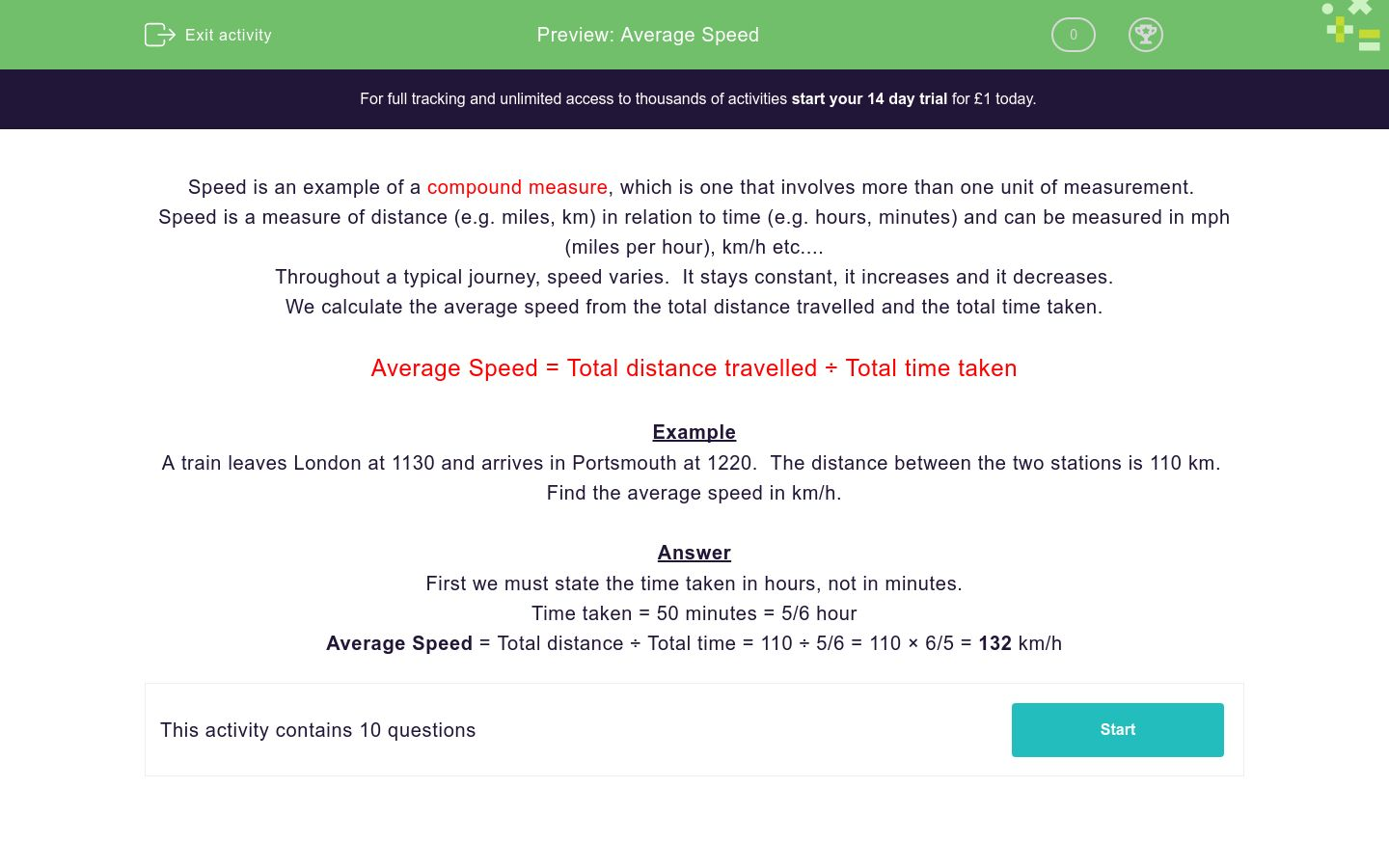# Average Speed

In this worksheet, students work out average speeds given the total distance travelled and the total time taken to complete the journey.Key stage:  KS 4

Curriculum topic:  Ratio, Proportion and Rates of Change

Curriculum subtopic:  Convert Between Related Compound Units

Difficulty level:### QUESTION 1 of 10

Speed is an example of a compound measure, which is one that involves more than one unit of measurement.

Speed is a measure of distance (e.g. miles, km) in relation to time (e.g. hours, minutes) and can be measured in mph (miles per hour), km/h etc....

Throughout a typical journey, speed varies.  It stays constant, it increases and it decreases.

We calculate the average speed from the total distance travelled and the total time taken.

Average Speed = Total distance travelled ÷ Total time taken

Example

A train leaves London at 1130 and arrives in Portsmouth at 1220.  The distance between the two stations is 110 km.  Find the average speed in km/h.

Answer

First we must state the time taken in hours, not in minutes.

Time taken = 50 minutes = 5/6 hour

Average Speed = Total distance ÷ Total time = 110 ÷ 5/6 = 110 × 6/5 = 132 km/h

A train leaves London at 1130 and arrives in Brighton at 1230.  The distance between the two stations is 80 km.

Find the average speed in km/h.

(just write the number)

A train travels 189 km from London to Bristol in 1½ hours.

Find the average speed in km/h.

(just write the number)

A car travels 144 miles in 2 hours.

Find the average speed in mph.

(just write the number)

A train travels 279 miles in 2¼ hours.

Find the average speed in mph.

(just write the number)

A car travels the 36 miles from Liverpool to Manchester in ¾ hour.

Find the average speed in mph.

(just write the number)

A car travels the 10.5 miles from Leeds to Bradford in 30 minutes.

Find the average speed in mph.

(just write the number)

A cyclist travels the 10.5 miles from Leeds to Bradford in 90 minutes.

Find the average speed in mph.

(just write the number)

A plane flies from London to Rome, a distance of 1503 km.  The flying time is 2¼ hours.

Find the average speed in km/h.

(just write the number)

A plane flies from London to New York, on a route that is 5452 km long.  The flying time is 7¼ hours.

Find the average speed in km/h.

(just write the number)

A bus leaves Birmingham at 11.10 a.m. and arrives in Rugby at 12.30 p.m.  The journey is 56 km long.

Find the average speed in km/h.

(just write the number)

• Question 1

A train leaves London at 1130 and arrives in Brighton at 1230.  The distance between the two stations is 80 km.

Find the average speed in km/h.

(just write the number)

CORRECT ANSWER
80
EDDIE SAYS
80 ÷ 1
• Question 2

A train travels 189 km from London to Bristol in 1½ hours.

Find the average speed in km/h.

(just write the number)

CORRECT ANSWER
126
EDDIE SAYS
189 ÷ 1½
• Question 3

A car travels 144 miles in 2 hours.

Find the average speed in mph.

(just write the number)

CORRECT ANSWER
72
EDDIE SAYS
144 ÷ 2
• Question 4

A train travels 279 miles in 2¼ hours.

Find the average speed in mph.

(just write the number)

CORRECT ANSWER
124
EDDIE SAYS
279 ÷ 2¼
• Question 5

A car travels the 36 miles from Liverpool to Manchester in ¾ hour.

Find the average speed in mph.

(just write the number)

CORRECT ANSWER
48
EDDIE SAYS
36 ÷ ¾
• Question 6

A car travels the 10.5 miles from Leeds to Bradford in 30 minutes.

Find the average speed in mph.

(just write the number)

CORRECT ANSWER
21
EDDIE SAYS
10.5 ÷ ½
• Question 7

A cyclist travels the 10.5 miles from Leeds to Bradford in 90 minutes.

Find the average speed in mph.

(just write the number)

CORRECT ANSWER
7
EDDIE SAYS
10.5 ÷ 1½
• Question 8

A plane flies from London to Rome, a distance of 1503 km.  The flying time is 2¼ hours.

Find the average speed in km/h.

(just write the number)

CORRECT ANSWER
668
EDDIE SAYS
1503 ÷ 2¼
• Question 9

A plane flies from London to New York, on a route that is 5452 km long.  The flying time is 7¼ hours.

Find the average speed in km/h.

(just write the number)

CORRECT ANSWER
752
EDDIE SAYS
5452 ÷ 7¼
• Question 10

A bus leaves Birmingham at 11.10 a.m. and arrives in Rugby at 12.30 p.m.  The journey is 56 km long.

Find the average speed in km/h.

(just write the number)

CORRECT ANSWER
42
EDDIE SAYS
56 ÷ 1⅓
---- OR ----

Sign up for a £1 trial so you can track and measure your child's progress on this activity.

### What is EdPlace?

We're your National Curriculum aligned online education content provider helping each child succeed in English, maths and science from year 1 to GCSE. With an EdPlace account you’ll be able to track and measure progress, helping each child achieve their best. We build confidence and attainment by personalising each child’s learning at a level that suits them.

Get started#### Similar activities you may like

Start your £1 trial today.
Subscribe from £10/month.